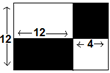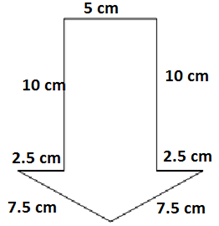# CREST Mathematics Olympiad Class 4 Sample Papers

## Syllabus:

Section 1: Numerals and Number Names, Number Sense (5-digit numbers), Computation Operations, Fractions, Length, Weight, Capacity, Time, Money, Geometry, Perimeter of Various Shapes, Symmetry, Conversions, Data Handling.

Achievers Section: Higher Order Thinking Questions - Syllabus as per Section 1

 Q.1 Simplify: 8 - 3 × (21 ÷ 7) + 10
 Q.2 LC - IX + D - XL =
 Q.3 The sum of first five prime numbers is:
 Q.4 The 18th multiple of 24 is ________.
 Q.5 The perimeter of the unshaded regions is _________.Q.6 Find the perimeter of the shape given in the following figure.Q.7 A scalene triangle is reshaped in such a way that it forms an isosceles triangle. What is the change in the perimeter?
 Q.8 I saw a Cobra snake in a zoo and asked a worker to estimate the length of the snake. He told me that it is seven times longer than a normal snake of length 2.5m. What is the length of the Cobra snake?
 Q.9 50 ones + 50 ones = One ____.
 Q.10 What must be subtracted from 54872 to obtain 13475?

Sample PDF of CREST Mathematics Olympiad for Class 4: